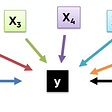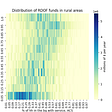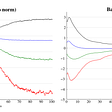# Diamond price prediction using Python on PriceScope and CaratLane diamond listings

I have downloaded the data for roughly 1500 diamonds for my own learning and not for any commercial purpose. The data is available on public domain on pricescope.com and is viewable and downloadable by anyone.

`df = pd.read_csv("pricescope1.csv")df.drop(’Unnamed: 0’, axis=1, inplace=True)display(df.head(3))`

## Data pre-processing

1. Replace \$ with blank using regex
2. Convert to int using astype()
3. Convert array / series to dataframe using pd.DataFrame
`priceint=pd.DataFrame(df[’price’].replace(’[\\$,]’, '’, regex=True).astype(int))df.drop([’price’], axis=1, inplace=True)df[’price’] = priceint[’price’].values`
`df.isnull().sum()`
`df[‘flr’].isnull().sum() / len(df[‘flr’]) *100`
`indexnames = df[df[’flr’].isnull()].indexdf.drop(axis=0,index=indexnames,inplace=True)`
`df.info()`
`<class 'pandas.core.frame.DataFrame'>Int64Index: 1409 entries, 6 to 1414Data columns (total 12 columns):carat      1409 non-null float64cut        1409 non-null objectcolor      1409 non-null objectclarity    1409 non-null objectdepth      1409 non-null objecttable      1409 non-null objectlab        1409 non-null objectsym        1409 non-null objectpol        1409 non-null objectflr        1409 non-null objecthna        1409 non-null objectprice      1409 non-null int32dtypes: float64(1), int32(1), object(10)memory usage: 137.6+ KB`
`lenT = len([x for x in df['table'] if not x.isnumeric()]) / len(df['table'])*100print('Percent of non-numeric data in Table -->', lenT)lenD = len([x for x in df['depth'] if not x.isnumeric()]) / len(df['depth'])*100print('Percent of non-numeric data in Depth -->', lenD)`
`all rubish values --> {'-'}Percent of identified rubbish data in Table --> 0.07097232079488999all rubish values --> {'-'}Percent of identified rubbish data in Depth --> 0.07097232079488999`
`indexnames = df[(df[‘table’] == ‘-’) | (df[‘depth’] == ‘-’)].indexdf.drop(axis=0,index=indexnames,inplace=True)`
`df[‘table’] = df[‘table’].astype(float)df[‘depth’] = df[‘depth’].astype(float)`
`sns.pairplot(df, x_vars=[‘carat’, ‘cut’, ‘clarity’, ‘color’], y_vars = [‘price’])plt.show()`

## Run ML algorithms

`X_df = df.drop([‘price’], axis=1)y_df = df[[‘price’]]`
`from sklearn.model_selection import train_test_splitX_train, X_test, y_train, y_test = train_test_split(X_df, y_df, test_size=0.3, random_state=42)`
`from sklearn.linear_model import LinearRegressionreg_all = LinearRegression()reg_all.fit(X_train,y_train)y_pred=reg_all.predict(X_test)`
`MAE: 0.11652136924155168MSE: 0.02292105733384392RMSE: 0.15139701890672722`
`MAE: 0.06791631018129721MSE: 0.010798208786551588RMSE: 0.10391443011705154`
`newdiamond = [‘0.3’, ‘Premium’, ‘ G’, ‘VS1’, 57, 516, ‘GIA’, ‘X’, ‘X’, ’N’, ‘N’]rf.predict(newdiamond)`

# References

--

--

--

## More from Jatin Grover

Data Engineer. Investor, blogger (https://fivestepguide.com) quadragenarian, father of 4 year old. I like to share knowledge & experience gained over 20+ years

Love podcasts or audiobooks? Learn on the go with our new app.

## Current Job Trends 2021## FRED API and Python## CLUSTERING DATA TO PREDICT LOAN APPROVAL## Linear Regression Types and Implementation!## Meet our team: Trouni, Data Science bootcamp manager## The #RDOF Is Progress in Closing the Digital Divide## Curse of Batch Normalization## What I Learned Writing my First Academic Article